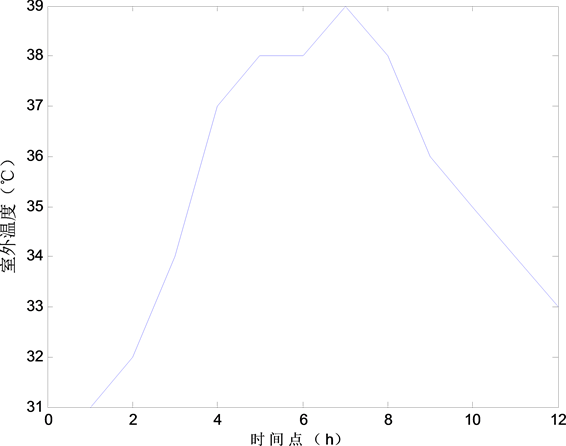# 基于建立虚拟电厂的水泥厂智能用电策略研究Study on Intelligent Power Consumption Strategy of Cement Plant Based on Virtual Power Plant

• 全文下载: PDF(645KB)    PP.160-167   DOI: 10.12677/SG.2018.82019
• 下载量: 418  浏览量: 903

As the automation degree of production process is increasing day by day, the coal consumption index of cement enterprises has come to decline year by year, but the electric power consumption still shows a trend of increasing with high electricity expenses. In this context, a mathematical model for the production electricity load based on adjustable equipment in cement plant is established. And the electric load modeling of temperature control equipment is taken into account when considering the environmental comfort degree of production area. On this basis, considering the user’s purchasing power cost, the optimal operation model of industrial intelligent power consumption is established. Finally, taking a cement plant in Yancheng, Jiangsu as an example, the economy of the optimized operation model of industrial intelligent power management is analyzed.

1. 引言

2. 基于可调节设备的生产用电负荷建模

2.1. 水泥生产流程

2.2. 负荷分析

2.3. 生产用电设备负荷模型

${x}_{i}^{t}=\left\{\begin{array}{l}1,\text{\hspace{0.17em}}\text{\hspace{0.17em}}\text{ }用电设备打开\\ 0,\text{\hspace{0.17em}}\text{\hspace{0.17em}}用电设备关闭\end{array}$

${p}_{t}^{p}=\underset{i=1}{\overset{n}{\sum }}{x}_{i}^{t}P\left(i\right)$ (1)

1) 等式约束

$\underset{t=1}{\overset{24}{\sum }}{x}_{i}^{t}\cdot \Delta t={T}_{i}$ (2)

2) 不等式约束

$\left\{\begin{array}{l}{\stackrel{¯}{P}}_{J}+{P}_{J}\le D\\ {\stackrel{¯}{P}}_{M}+{P}_{M}\le D\\ {\stackrel{¯}{P}}_{0}+{P}_{0}\le D\\ {\stackrel{¯}{P}}_{m}+{P}_{m}\le D\end{array}$ (3)3. 计及舒适度的温控设备用电负荷建模

3.1. 空调能量消耗模型

${P}_{i,t}=\frac{{T}_{i.t}-{T}_{i,t-1}-\alpha \left({T}_{out\text{_}t}-{T}_{i,t-1}\right)}{{\beta }_{i}}$ (4)

${p}_{t}=\underset{i=1}{\overset{m}{\sum }}{P}_{i,t}$ (5)

3.2. 热舒适指标的选取

$\text{PMV}=\left(0.32{\text{e}}^{-0.042M/A}+0.032\right)\cdot L$ (6)

PMV的取值范围是−3~+3，分别对应了人体的冷感觉和热感觉，如图1所示，PMV将人体的热感觉分为7个层次，当室内PMV值在[−1,+1]以内时，人体感觉较为舒适，所以在人体舒适的范围内，制冷时提高PMV的设置值和制热时降低PMV的设置值可以降低空调负荷和节约能源  。

3.3. 虚拟电厂的模型Figure 1. Comfort index PMV and energy saving

${p}_{t}^{b}={p}_{t}^{-}-{p}_{t}^{+}$ (7)

$\begin{array}{l}0\le {P}_{t}^{-}\le {P}_{\mathrm{max}}\\ 0\le {P}_{t}^{+}\le {P}_{\mathrm{max}}\end{array}$ (8)

$-1\le \text{PMV}\le 1$ (9)

4. 智能用电管理的数学模型

4.1. 目标函数

$\mathrm{min}\text{\hspace{0.17em}}{V}_{\text{cost}}=\underset{t=1}{\overset{n}{\sum }}{\lambda }_{t}{p}_{t}^{a}\cdot \Delta t+\underset{t=1}{\overset{n}{\sum }}\gamma {p}_{t}^{b}\cdot \Delta t$ (10)

4.2. 约束条件

1) 电功率平衡约束

${p}_{t}^{p}={p}_{t}^{a}+{p}_{t}^{b}$ (11)

2) 发电机组出力约束

${P}_{\mathrm{min},i}\le {P}_{i}^{t}{U}_{i}^{t}\le {P}_{\mathrm{max},i},\text{\hspace{0.17em}}\text{\hspace{0.17em}}t=1,\cdots ,T;\text{\hspace{0.17em}}i\in N$ (12)

3) 基于可中断负荷的生产用电约束，如式(2)和式(3)。

4) 计及舒适度的温控设备用电约束，如式(8)和式(9)。

5. 算例分析

5.1. 算例数据Table 2. Peak and valley period and electricity price in a cement plant5.2. 用电情况分析Figure 2. The temperature on the day of predictionTable 4. The electric order of the optimized industrial interruptible loadTable 5. The output of virtual power plant produced by air conditioning energy savingTable 6. Electricity cost under different power consumption conditions

6. 结语

  张宁. 水泥生产电耗预测与用电负荷优化调度技术研究[D]: [硕士学位论文]. 天津: 天津理工大学, 2015.  刘松辉, 汪澜, 王俊杰. 水泥厂粉磨设备用电模式研究[J]. 水泥CEMENT, 2014(12): 31-32.  代家强. 面向工商业负荷的智能用电能量管理建模及优化研究[D]: [硕士学位论文]. 天津: 天津大学, 2013.  陈俊生. 面向智能用电的需求响应技术及家庭用户用电策略研究[D]: [硕士学位论文]. 重庆: 重庆大学, 2014.  Dang, J. and Harley, R.G. (2012) Air Conditioner Optimal Scheduling Using Best Response Techniques. Power Engineering Society Conference and Exposition in Africa, Johannesburg, 1-5. https://doi.org/10.1109/PowerAfrica.2012.6498655  Yonezawa, K., Yamada, F., et al. (2000) Comfort Air-Conditioning Control for Building Energy-Saving. Proceedings of the 26th Annual Conference of the IEEE Industrial Electronics Society, 3, 1737-1742.  Sato, K., Samejima, M., et al. (2012) A Scheduling Method of Air Conditioner Operation Using Workers Daily Ac-tion Plan towards Energy Saving and Comfort at Office. Emerging Technologies & Factory Automation, 17, 1-6.  张清琳. 温度控制与热舒适控制空调系统舒适性及节能性比较研究[D]: [硕士学位论文]. 长沙: 湖南大学, 2012.  陈少梁. 虚拟电厂的负荷优化调度研究[D]: [硕士学位论文]. 北京: 华北电力大学(北京), 2016.  于娜, 于乐征, 朱景明. 峰谷分时电价下过程工业用户的用电响应模型[J]. 东北电力大学学报, 2014, 34(4): 17-21.  姚玉玺. 基于遗传算法的校园空调节能方法研究[D]: [硕士学位论文]. 杭州: 杭州电子科技大学, 2014.